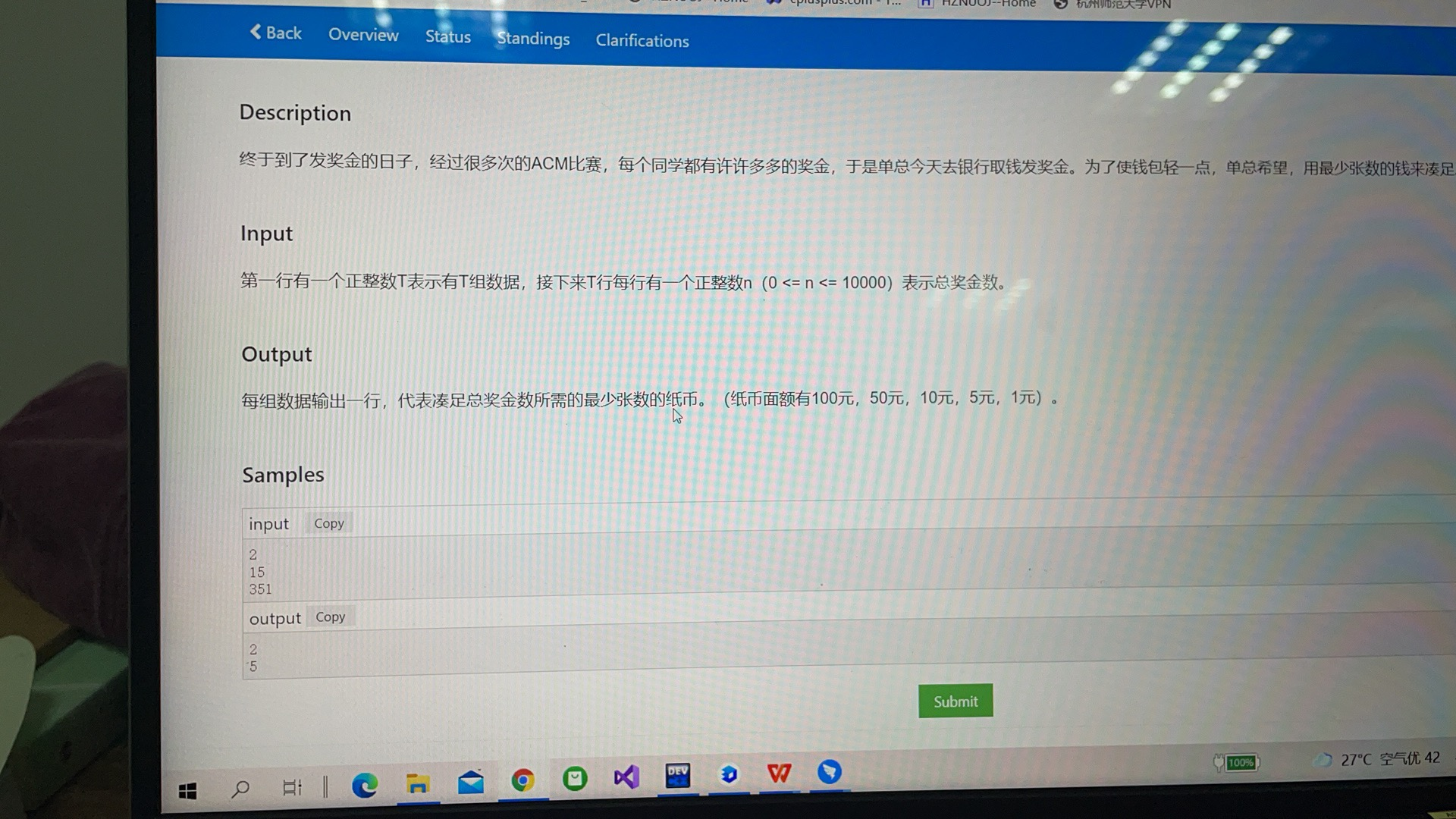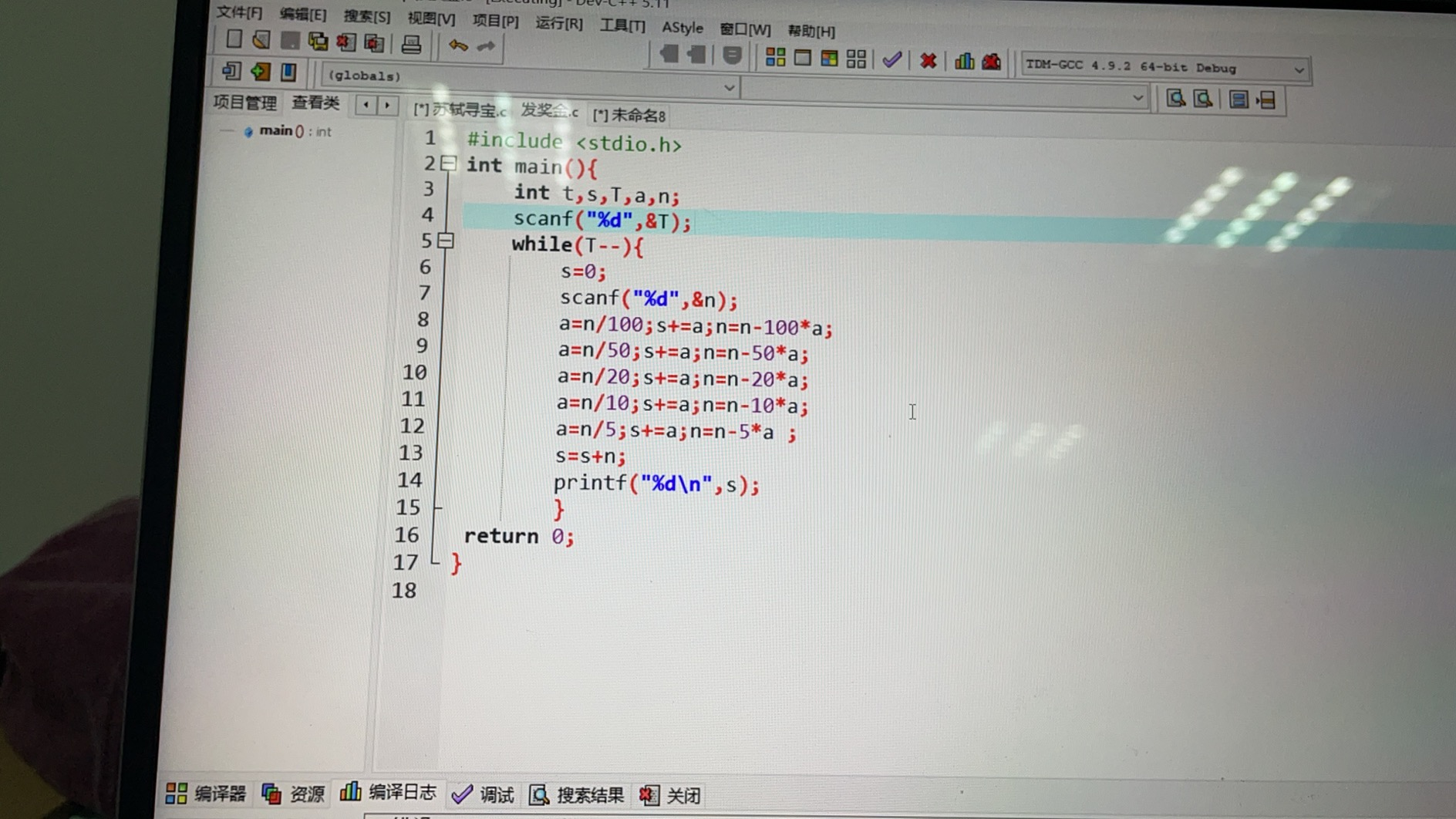「已注销」
2021-10-06 18:28#include <stdio.h>
int main(){
int t,s,T,a,n;
scanf("%d",&T);
while(T--){
s=0;
scanf("%d",&n);
a=n/100;s+=a;n=n-100a;
a=n/50;s+=a;n=n-50
a;
a=n/20;s+=a;n=n-20a;
a=n/10;s+=a;n=n-10
a;
a=n/5;s+=a;n=n-5*a ;
s=s+n;
printf("%d\n",s);
}
return 0;
}

• 写回答
• 好问题 提建议
• 追加酬金
• 关注问题
• 收藏
• 邀请回答